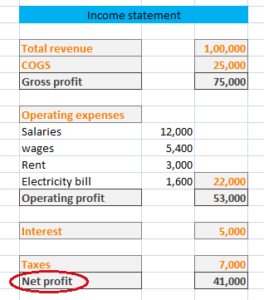# Net profit – Meaning & Formula

Net profit is the excess of a company’s net revenue (net sales) over its total expenses. For this purpose, total expenses include both direct and indirect expenses as well as interest and taxes.

If a company is liable to pay dividend to preference shareholders then the amount of dividend is also subtracted to get this figure. Here, it should be noted that the dividend paid to equity shareholders is not deducted from net sales.

Net profit is the also known as Profit after tax (PAT).

### Formula for the calculation of net profit:

Net sales – COGS* – Operating expenses – Interest and taxes – Dividend to preference shareholders (If any)

Or

Gross profit – Operating expenses – Interest and taxes – Dividend to preference shareholders (If any)

Or

Operating profit – Interest and Taxes – Dividend to preference shareholders (If any)

*Cost of goods sold (COGS) = Opening stock + Purchases – Closing stock

### Example:

From the figures provided in income statement of XYZ ltd, PAT can be calculated as:Net profit = Gross profit – Operating expenses – Interest and taxes

= 75,000 – 22,000 – 12,000* = 41,000

Or

Operating profit – (Interest and Taxes)

= 53,000 – 12,000* = 41,000

* The given figure is the sum total of Interest and Taxes.